##### Trigonometry Workbook For DummiesEven though each trigonometry function is perfectly wonderful, being able to express each trig function in terms of one of the other five trig functions is frequently to your advantage. For example, you may have some sine terms in an expression that you want to express in terms of cotangent, so that all the functions match, making it easier to solve the equation.

To write the sine function in terms of cotangent, follow these steps:

1. Start with the ratio identity involving sine, cosine, and tangent, and multiply each side by cosine to get the sine alone on the left.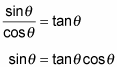2. Replace cosine with its reciprocal function.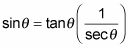3. Solve the Pythagorean identity tan2θ + 1 = sec2θ for secant.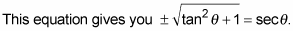4. Replace the secant in the sine equation.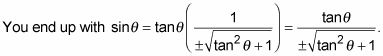5. Replace all the tangents with 1 over the reciprocal for tangent (which is cotangent) and simplify the expression.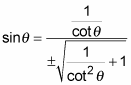The result is a complex fraction — it has fractions in both the numerator and denominator — so it’ll look a lot better if you simplify it.

6. Rewrite the part under the radical as a single fraction and simplify it by taking the square root of each part.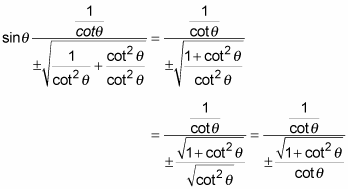7. Multiply the numerator by the reciprocal of the denominator.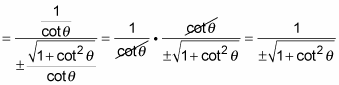Voilà — you have sine in terms of cotangent.